# Phase-field mobility for crystal growth rates in undercooled silicates, SiO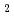and GeOliquids
Phase-field mobility,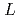, and crystal growth rates in crystallization of 11 oxides or mixed oxides in undercooled silicates, SiOand GeOliquids were calculated with a simple phase-field model (PFM), and material dependence of thewas discussed. Ratios between experimental crystal growth rates and the PFM simulation with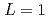were confirmed to be proportional to a power of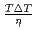on the solid/liquid interface process during the crystal growth in a log-log plot. We determined that parameters,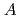and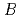, of the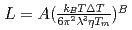were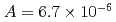to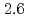m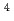J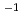sand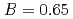to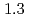, which were unique for the materials. It was confirmed that our PFM simulation with the determinedreproduced quantitively the experimental crystal growth rates. Thehas a proportional relationship with the diffusion coefficient of a cation molar mass average per unit an oxygen molar mass at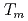in a log-log graph. Thedepends on the sum of the cation molar mass per the oxygen molar mass,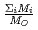, in a compound. In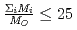, thedecreases with the cation molar mass increasing. The assumed cause is that the B represents the degree of the temperature dependence of the. Since the cation molar mass is proportional to an inertial resistance of the cation transfer, thedecreases with inverse of the cation molar mass. In crystallization of the silicates of heavy cation in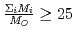, thesaturates at approximately 0.67, which leads to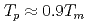.